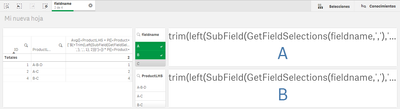Qlik Sense App Development

Discussion board where members can learn more about Qlik Sense App Development and Usage.

AnnouncementsPartner

How to use many selections of a field to filter another field.

Hi,

I've done a model with Association Rules with my Field Products {A, B, C, D, E}.

So, for exemple i've obtained the rule  {A,C}-->{E} that means that if you have bought A and C, normally you will also buy the E.

In Qlik the table is like:The relationship between them is: if a click on Product 'A' the result in table must be rows with 'A-B-D' and 'A-C' because they contain 'A'. That works good. The problem is that my goal would by to click in more than one product and obtain only the rules with this products in the same row. For example, if I click on 'A' and 'B' of Products, the only row that should be shown is first one: A-B-D. But qlik by default selects the rowns tha contain 'A' or 'B', like 'A-B-D', 'A-C' and 'B-C'.

I've got the objetive manually with:

Avg({<ProductLHS = P({<Product={'A'}>})*P({<Product={'B'}>})>} ID)

But I need that this filter with 'A' or 'B' depend on selections of Product. With

trim(left(SubField(GetFieldSelections(fieldname,','),',',1),2))

trim(left(SubField(GetFieldSelections(fieldname,','),',',2),2))

I obtain the selections, but when I introduce it on the Set Analysis of measure it doesnt recognize the selections.

Does anybody know how to fix it? Thanks!!

Labels (3)

• qliksense filter

1 Solution

Accepted SolutionsMVP

Re: How to use many selections of a field to filter another field.

I would simplify the expression to this

Avg({<ProductLHS = P({<fieldname={"\$(=If(Len(Trim(SubField(GetFieldSelections(fieldname,','),',',1))) = 0, '*', Trim(SubField(GetFieldSelections(fieldname,','),',',1))))"}>}) *
P({<fieldname={"\$(=If(Len(Trim(SubField(GetFieldSelections(fieldname,','),',',2))) = 0, '*', Trim(SubField(GetFieldSelections(fieldname,','),',',2))))"}>}) *
P({<fieldname={"\$(=If(Len(Trim(SubField(GetFieldSelections(fieldname,','),',',3))) = 0, '*', Trim(SubField(GetFieldSelections(fieldname,','),',',3))))"}>}) *
P({<fieldname={"\$(=If(Len(Trim(SubField(GetFieldSelections(fieldname,','),',',4))) = 0, '*', Trim(SubField(GetFieldSelections(fieldname,','),',',4))))"}>})
>} ID)

Basically checking for each selection if there is a selection... if there is no selection replace the Product with * which means include everything....

Note: * will exclude nulls in fieldname... so make sure there are no nulls....

8 RepliesMVP

Re: How to use many selections of a field to filter another field.

Are you saying that this doesn't work for you?

Avg({<ProductLHS = P({<Product={"\$(=Trim(Left(SubField(GetFieldSelections(fieldname, ','), ',', 1), 2)))"}>}) * P({<Product={"\$(=Trim(Left(SubField(GetFieldSelections(fieldname, ','), ',', 2), 2)))"}>})>} ID)Partner

Re: How to use many selections of a field to filter another field.

Yes,and if I put  Avg({<ProductLHS = P({<fieldname={'A'}>})*P({<fieldname={'B'}>})>} ID) it works:MVP

Re: How to use many selections of a field to filter another field.

What is the different between fieldname and Product? Are you able to share a sample qvf file to check this out?Partner

Re: How to use many selections of a field to filter another field.

Oh Sorry,

fieldname and Product are the same.

I put here my example app.

Thanks.MVP

Re: How to use many selections of a field to filter another field.

This seems to work

Avg({<ProductLHS = P({<fieldname={"\$(=Trim(Left(SubField(GetFieldSelections(fieldname, ','), ',', 1), 2)))"}>}) * P({<fieldname={"\$(=Trim(Left(SubField(GetFieldSelections(fieldname, ','), ',', 2), 2)))"}>})>} ID)

Only change that I made was to change the field name Product to fieldname here...Partner

Re: How to use many selections of a field to filter another field.

Yes! It works!

So finally, if I want that show only cases based on the four posible selections in this case, how do I have to do it?

For example,

• No selections: show me all possible values
• One filter: show me case with this value
• Two filters: show me cases with both values
• Etc

I mean, extend this to all possible cases. I'm testing with:

if(GetSelectedCount(fieldname)=1,
Avg({<ProductLHS = P({<fieldname={"\$(=Trim(Left(SubField(GetFieldSelections(fieldname, ','), ',', 1), 2)))"}>})>} ID),
if(GetSelectedCount(fieldname)=2,
Avg({<ProductLHS = P({<fieldname={"\$(=Trim(Left(SubField(GetFieldSelections(fieldname, ','), ',', 1), 2)))"}>}) * P({<fieldname={"\$(=Trim(Left(SubField(GetFieldSelections(fieldname, ','), ',', 2), 2)))"}>})>} ID),
if(GetSelectedCount(fieldname)=3,
Avg({<ProductLHS = P({<fieldname={"\$(=Trim(Left(SubField(GetFieldSelections(fieldname, ','), ',', 1), 2)))"}>}) * P({<fieldname={"\$(=Trim(Left(SubField(GetFieldSelections(fieldname, ','), ',', 2), 2)))"}>})* P({<fieldname={"\$(=Trim(Left(SubField(GetFieldSelections(fieldname, ','), ',', 3), 2)))"}>})>} ID),
if(GetSelectedCount(fieldname)=4,
Avg({<ProductLHS = P({<fieldname={"\$(=Trim(Left(SubField(GetFieldSelections(fieldname, ','), ',', 1), 2)))"}>}) * P({<fieldname={"\$(=Trim(Left(SubField(GetFieldSelections(fieldname, ','), ',', 2), 2)))"}>})* P({<fieldname={"\$(=Trim(Left(SubField(GetFieldSelections(fieldname, ','), ',', 3), 2)))"}>})* P({<fieldname={"\$(=Trim(Left(SubField(GetFieldSelections(fieldname, ','), ',', 4), 2)))"}>})>} ID)
))))

but it doesn't work....MVP

Re: How to use many selections of a field to filter another field.

I would simplify the expression to this

Avg({<ProductLHS = P({<fieldname={"\$(=If(Len(Trim(SubField(GetFieldSelections(fieldname,','),',',1))) = 0, '*', Trim(SubField(GetFieldSelections(fieldname,','),',',1))))"}>}) *
P({<fieldname={"\$(=If(Len(Trim(SubField(GetFieldSelections(fieldname,','),',',2))) = 0, '*', Trim(SubField(GetFieldSelections(fieldname,','),',',2))))"}>}) *
P({<fieldname={"\$(=If(Len(Trim(SubField(GetFieldSelections(fieldname,','),',',3))) = 0, '*', Trim(SubField(GetFieldSelections(fieldname,','),',',3))))"}>}) *
P({<fieldname={"\$(=If(Len(Trim(SubField(GetFieldSelections(fieldname,','),',',4))) = 0, '*', Trim(SubField(GetFieldSelections(fieldname,','),',',4))))"}>})
>} ID)

Basically checking for each selection if there is a selection... if there is no selection replace the Product with * which means include everything....

Note: * will exclude nulls in fieldname... so make sure there are no nulls....Partner

Re: How to use many selections of a field to filter another field.

Yes, this is the finally solution.

Thank's a lot Sunny 🙂  You're the real MVP!!!!!!